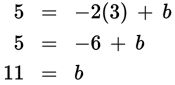# SAT Math Multiple Choice Question 478: Answer and Explanation

### Test Information

Question: 478

13. Ramon graphed a line that has a slope of –2. The line he graphed passes through the point (3, 5). If Ramon doubles the slope of his line and then shifts it down 1 unit, through which point will the line pass?

• A. (3, –2)
• B. (3, 9)
• C. (6, 4)
• D. (10, 2)

Explanation:

A

Difficulty: Hard

Category: Heart of Algebra / Linear Equations

Strategic Advice: You do not have enough information to answer this question using rules of transformations. Instead, you'll need to write the equation of the original line, then follow the criteria given to change the equation and find the point.

Getting to the Answer: You already know the slope of the original line (m = –2), but you need the y-intercept. You also know a point through which the line passes. Plug the x- and y-values of this point into slope-intercept form of a line, and solve for b.The equation of the original line is y = –2x + 11. If Ramon doubles the slope, it goes from –2 to 2(–2) = –4. If he shifts the line down one unit, the y-intercept becomes 11 – 1 = 10, so the equation of the new line is y = –4x + 10. Graph the line in your graphing calculator and see that it passes through the point (3, –2). You could also plug the x- and y-values of the points in the answer choices into the new equation to see which one results in a true statement, but this could take more time depending on which choice is correct on Test Day.